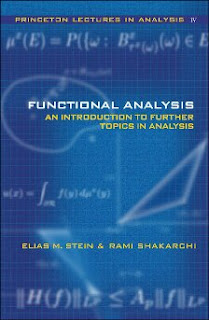Functional Analysis : Introduction to Further Topics in Analysis

### PREFACE :

This is the fourth and final volume in the Princeton Lectures in Analysis, a series of textbooks that aim to present, in an integrated manner, the core areas of analysis. Beginning with the basic facts of functional analysis, this volume looks at Banach spaces, Lp spaces, and distribution theory, and highlights their roles in harmonic analysis. The authors then use the Baire category theorem to illustrate several points, including the existence of Besicovitch sets. The second half of the book introduces readers to other central topics in analysis, such as probability theory and Brownian motion, which culminates in the solution of Dirichlet's problem. The concluding chapters explore several complex variables and oscillatory integrals in Fourier analysis, and illustrate applications to such diverse areas as nonlinear dispersion equations and the problem of counting lattice points. Throughout the book, the authors focus on key results in each area and stress the organic unity of the subject. -A comprehensive and authoritative text that treats some of the main topics of modern analysis -A look at basic functional analysis and its applications in harmonic analysis, probability theory, and several complex variables -Key results in each area discussed in relation to other areas of mathematics -Highlights the organic unity of large areas of analysis traditionally split into subfields -Interesting exercises and problems illustrate ideas -Clear proofs provided